# ISEE Middle Level Quantitative : Mean

## Example Questions

← Previous 1 3 4 5

### Example Question #1 : Mean

Brandon is training for a marathon.  Each day after school he runs for two hours.  Over the course of two weeks, he runs the following distances: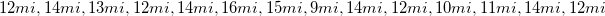What is the mean distance Brandon runs in this two week period?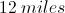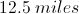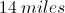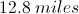Explanation:

Since the mean is the average of the data, we must calculate the average of Brandon's runs. In order to do this, we first add up all the data points.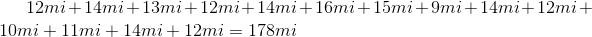Next we divide by the total number of days that Brandon runs,.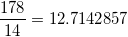We can round this to the nearest tenth,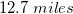### Example Question #2 : Mean

Using the information given in each question, compare the quantity in Column A to the quantity in Column B.

Allie has scored 79, 77, 96, 71, 85, 86, 90, and 84 on her eight science quizzes, and her teacher drops the highest and lowest score.

Column A                                 Column B

Allie's quiz average                   Allie's quiz average

before the dropped scores         after the dropped scores

The two quantities are equal.

The quantity in Column B is greater.

The relationship cannot be determined from the information given.

The quantity in Column A is greater.

The two quantities are equal.

Explanation:

Add up the 8 test scores and divide by 8 to figure out the mean (83.5). Now figure out the average between the highest and lowest scores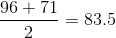Since the dropped tests have the same average as the original 8 tests, Allie's score would not change. The correct answer is that the two columns are equal.

Note: If you are good at mental math, here is a quick way to figure out the average of the 8 tests:

First, estimate the average (say, 80). Next, go through the numbers and add or subtract the difference between the number and 80.

79 is 1 below 80.

77 is 3 below 80 (so now we're 4 below 80).

As we continue through the numbers, we get

12 above, 3 above, 8 above, 14 above, 24 above, 28 above.

So the 8 scores are cumulatively 28 above an average score of 80. Now, simply divide 28 by 8 and add this to 80.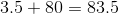### Example Question #3 : Mean

A student's grade in Professor Kalton's abstract algebra class is the mean of his or her five test scores.

Kent's five scores are 83, 91, 88, 85, and 80.

Janice's five scores are 87, 87, 84, 85, and 92.

Which is the higher quantity?

(a) and (b) are equal

(b) is greater

(a) is greater

It is impossible to tell from the information given

(b) is greater

Explanation:

You do not need to take the two means; just add the scores, since each sum will be divided by 5 to get the students' means.

Kent's total: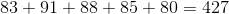Janice's total: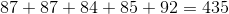Janice's sum is higher; so is her mean score.

### Example Question #3 : How To Find Mean

The sum of the weights of the twelve students in the math club is 1,980 pounds. Which is the greater quantity?

(A) The mean of their weights

(B) 175 pounds

(B) is greater

(A) is greater

(A) and (B) are equal

It is impossible to determine which is greater from the information given

(B) is greater

Explanation:

To get the mean of the students' weights, divide the sum of the weights by the number of students; here, this is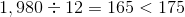(B) is greater.

### Example Question #1 : Mean

Consider the data set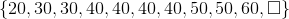Which of the following elements replaces the box to make 42 the mean of the data set?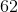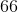Explanation:

For the mean of the eleven data elements to be 42, their sum divided by 11 must be 42. Equivalently, the sum of the elements must be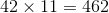The sum of the ten known elements is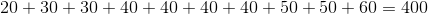Therefore, the unknown element must be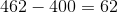### Example Question #1 : Mean

The six students in the science club weigh 145 pounds, 172 pounds, 166 pounds, 159 pounds, 153 pounds, and 201 pounds. Find the mean of their weights.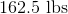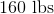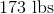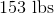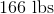Explanation:

Add the weights, then divide the sum by six: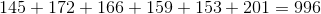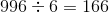### Example Question #2 : Mean

Give the mean of the data set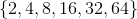Explanation:

There are six elements, so to find the mean, add the elements and divide by six: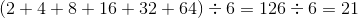### Example Question #8 : Mean

The mean of the weights of the eleven students in the math club is 186 pounds. Which is the greater quantity?

(A) The sum of the weights of the students

(B) One ton

(A) and (B) are equal

It is impossible to determine which is greater from the information given

(B) is greater

(A) is greater

(A) is greater

Explanation:

One ton is equal to 2,000 pounds.

The mean of the weights of the students is the total of their weights divided by the number of students.

Let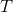be the total of their weights.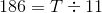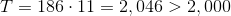(A) is greater.

### Example Question #281 : Isee Middle Level (Grades 7 8) Quantitative Reasoning

David scored 77, 92, and 80 on his first 3 tests. He currently has a test score average of 83. What grade would David need on the next test to increase his test score average to 85?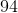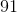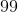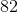Explanation:

You can find the average of a set of numbers by adding up the numbers and dividing by how many numbers are in the data set. Since David is trying to acheive an average of 85 by his fourth test, we should multiply 85 by 4.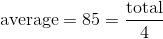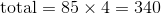The result is the number that the four tests should total in order to achieve an average of 85. Then, add up the test scores Daid has recieved so far: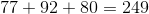If you subtract this number (the total from the test scores so far) from 340, the result will be the score needed to achieve the 85 average.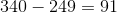David must score a 91 on his fourth test.

### Example Question #4 : Mean

June ran 2 miles Monday, 3 miles Tuesday, 3 miles Thursday, and 2 miles Friday. How many miles did June run on average from Monday to Friday?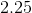Explanation:

You can find the average of a set of numbers by adding up all the numbers in that set and dividing by how many numbers you added total. Here is a summary of our set:

Monday: 2 miles

Tuesday: 3 miles

Wednesday: 0 miles

Thursday: 3 miles

Friday: 2 miles

Keep in mind that we are looking for the average number of miles June ran Monday through Friday, and that there are 5 days in that period. You must also account for the 0 miles that June ran on Wednesday.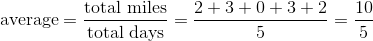June ran a total of 10 miles during that 5 day period. You must then divide by the number of days in order to get the average.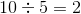← Previous 1 3 4 5

### All ISEE Middle Level Quantitative Resources